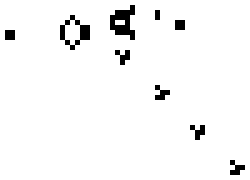# Functional Game of Life part 1 (C#)I thought it might be interesting to solve the Conway’s Game of Life cellular automaton in a functional manner. Game of life is an interesting problem for experimentation because it is simple and well understood.

## Where to Start?

I’ll start by defining a way to render the world. I want to start here because it is useful to have a nice way to visualise the state of the system, also it conveniently forces me to define my data model. I will model the system as a collection of living cells, each with X and Y coordinates (zero based). The living cells define the boundaries of the system. Dead cells are implicitly the cells within the system boundary that are not living. A system defined as:

``````new Cell(1,0), new Cell(0,1), new Cell(2,1), new Cell(7,16)
``````

will produce the following output (living cells are darker):

To get to this I started with a test (using giv.n and nunit).

``````[TestFixture]
public class RenderingTests
{
private List<Cell> _cells;
private string _result;

[Test]
public void RenderASmallSystem()
{
Giv.n(ASmallSystem);
Wh.n(ItIsRendered);
Th.n(() => ItShouldRenderDimensions(8, 17))
.And(() => LivingCellCountIs(_cells.Count));
}

private void ASmallSystem()
{
_cells = new List<Cell> { new Cell(1,0), new Cell(0,1), new Cell(2,1), new Cell(7,16) };
}

private void ItIsRendered()
{
_result = GameOfLife.Render(_cells);
}

private void ItShouldRenderDimensions(int x, int y)
{
Assert.AreEqual(x*y, CountOccurances(_result,"<div"));
}

private void LivingCellCountIs(int count)
{
Assert.AreEqual(count, CountOccurances(_result,"on"));
}

private static int CountOccurances(string source, string sub)
{
return source.Select((c, i) => source.Substring(i)).Count(s => s.StartsWith(sub));
}
}
``````

and the implementation so far:

``````public class GameOfLife
{
public static string Render(List<Cell> cells)
{
var largestX = cells.Max(c => c.X);
var largestY = cells.Max(c => c.Y);
Func<int,int,bool, string> buildCell = (x,y,on) =>
string.Format("<div class=\"cell {2}\" style=\"left: {0}px; top: {1}px;\">&nbsp;</div>", 20 * (x+1), 20 * (y+1), @on ? "on" : "");

var results = from x in Enumerable.Range(0, largestX+1)
from y in Enumerable.Range(0, largestY+1)
select buildCell(x, y, IsOn(cells, x, y));
return string.Join("\n", results);
}

private static bool IsOn(IEnumerable<Cell> cells, int x, int y)
{
return cells.Any(c => c.X == x && c.Y == y);
}
}

public struct Cell
{
public int X;
public int Y;

public Cell(int x, int y)
{
X = x;
Y = y;
}
}
``````

## Source

The source for this article is in a public git repo, under the tag `data-and-render`.

## Next

The next post in this series will look at randomly seeding the system with living cells, so that the cellular evolution can be started.